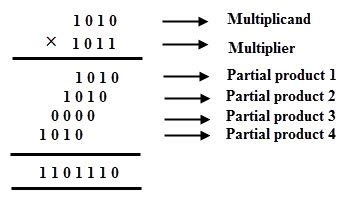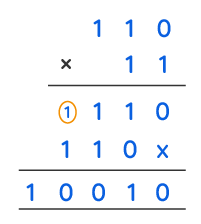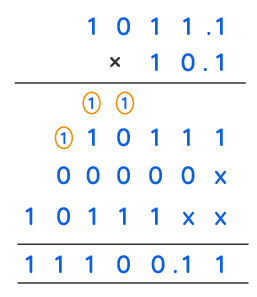# Binary Multiplication- the rules, uses

Of course, we all know that the computer does not understand our language at all. In fact, it only understands the language of 0’s and 1’s.

We call this the binary language.In fact, you can represent almost everything via 0’s and 1’s. So, in this article we shall discuss the binary Multiplication process in detail.

## Binary Multiplication rules

The process of multiplying binary numbers is known as binary multiplication. Also, the process of finding the product of binary numbers is the same as multiplying decimal values. However, the sole change is that binary multiplication works with numbers made up of 0s and 1s, whereas decimal multiplication works with numbers made up of digits ranging from 0 to 9. Now, we shall discuss the binary multiplication process one step at a time.

Multiplication of binary numbers is the same as multiplication of decimal numbers. A multiplier and a multiplicand are both present. Of course, the multiplication yields a result, which is a product. Because binary multiplication uses just binary digits, we can only multiply 0s and 1s. Now, we will focus on the rules for binary multiplication.

 Multiplicator Multiplicand Product 0 1 0 1 0 0 0 0 0 1 1 1

So, this is what such binary multiplication looks like.Because binary numbers have two digits that are either 0 or 1, multiplying binary numbers is easier than multiplying decimal numbers. This table explains how to multiply binary numbers. These conditions apply to binary integers with a percentage point as well.

Two digits, 0 and 1 represent any number in the binary system. As a result, the binary system’s base is two. Individual digits 0 and 1 represent the indices of powers of two in this system. The decimal number six, for example, is written as:

6= 4+2= 1×2^2+1×2^1+0×2^0. As a result, the decimal number 6 has a binary representation of 110.

## Binary multiplication worksheet

Of course, binary numbers are simply numbers made of 0’s and 1’s. So, finding their product is actually very easy. However, you need to have a little practice for it. Otherwise, you might end up making too many menial mistakes as well.

Here, we will show you a few basic examples of binary multiplication.

For example, you might want to find the product of:

(110)2 and (11)2.

So, in order to get this result, we have to follow the table closely.

We know that the rules for binary multiplication are:

0 × 0 = 0

0 × 1 = 0

1 × 0 = 0

1 × 1 = 1

Now, let us use these rules to multiply the binary numbers.

GIven, multiplicand = (110)2, multiplier =(11)2

However, the real process looks something like this:Now, we shall move on to the next example.

Find the product of (11011)2 and (101)2.

Here, multiplicand = (11011)2 and multiplier = (101)2.

So, you just need to follow the rules in the table above to obtain the desired result. Actually, it is pretty simple.

Also, the entire process looks like this:So, for the next example let us consider a binary number with decimal points. Even here, the process is quite the same actually.

For instance, multiply (1011.1)2 and (10.1)2 to find the result.

So, use the similar rules in the table to derive this result.Thus multiplying the binary numbers 1011.1 and 10.1 gives us 11100.11 to the base 2.

## Binary multiplication calculator

Doing the binary multiplication is pretty easy. However, you can use a calculator if you find any problem in doing it.

Binary multiplication calculator is an easy and free internet utility.

It has unique benefits:

• It gives very precise results and is quite easy to obtain.
• When compared to the pen-and-paper method, it takes a very little amount of the time.
• For the ‘binary multiplication’ method, you do not need to keep in mind the previously shown formula table.

The binary number system is a number system that works almost similar to the decimal system. And, most people are familiar with that. The binary number system employs 2 as its base. On the other hand, the decimal number system uses 10. Moreover, while the decimal system uses digits 0 to 9, the binary system only uses 0 and 1. Also, people refer to each digit as a bit. Aside from these changes, you can do operations like addition, subtraction, multiplication, and division using the decimal system’s rules.

The binary system is in use in almost all recent technology and computers. Mainly, it is due to its ease of application in digital circuits with logic gates. Or, in hardware that just has to discern two states, on and off (or true/false, present/absent, etc.) is far easier to design. Using a decimal system would need electronics able to detect ten states for all the digits from 0 to 9, as well as being harder.

So, you might want to use the binary multiplication calculator very often. We will discuss the steps to use this calculator in detail in the next part of the article.

## Binary multiplication converter

In order to perform binary multiplications, you also need to know the way to convert decimal numbers to binary as well.

The steps to make that conversion are:

• Within the given number, find the bigger power of two.
• Subtract that amount from the total.
• Within the leftover from step 2, find the larger power of two.
• Repeat until there are no more remains.
• For every binary place value that you got, enter a 1; for the rest, enter a 0.

Binary multiplication is a lot easier than decimal multiplication. Because 0 and 1 are the only values used, the outcomes that must be summed are either just like the first term or 0. As in decimal multiplication, placeholder 0’s must be added in each next row, and you must move the value to the left. Binary multiplication’s difficulty stems from lengthy binary addition based on the number of bits in each term.

However, follow these steps to perform the multiplication using the binary multiplication calculator:

Firstly, in the first box enter the multiplicand.

Secondly, enter the multiplicator in the second box.

Thirdly, choose the operator from the drop down in the middle. Choose the addition, subtraction, multiplication or division operator according to your need.

Finally, click on calculate.

So, that should give you your result.

Here, we shall link the calculator for you to use. However, you can use any other version of it as they are very easily found online.

## Binary multiplication algorithm

Booth’s multiplication algorithm is a two’s complement multiplication method that multiplies two signed binary numbers. Andrew Donald Booth devised the algorithm in 1950. In the field of computer architecture, Booth’s algorithm finds its use.

In signed two’s complement representation, Booth’s algorithm shows adjacent pairs of bits of the ‘N’-bit multiplier Y. Also, it includes an inferred bit below the least significant bit, y1 = 0. The bits yi and yi1 are considered for each bit yi, for I ranging from 0 to N 1. However, the product accumulator P remains intact when these two bits are equivalent. The multiplicand times 2i is added to P where yi = 0 and yi1 = 1. And, the multiplicand times 2i is deleted from P where yi = 1 and yi1 = 0. The signed product is the final value of P.

The multiplicand and product have no unique format. In fact, they are both represented in two’s complement. However, any number system that allows basic arithmetic will work. The timing of the stages is not defined, as mentioned here.

Starting at I = 0, it normally moves from LSB to MSB; the multiplication by 2i is then superseded by gradual shift of the P accumulator to the right between stages. Thus, low bits can be moved out, and further additions and subtractions can be done only on the highest N bits of P.

Read Also:Derivative of ln x – Natural Logarithm

In the multiplier, the method is generally stated as turning strings of 1s to a high-order +1 and a low-order 1 at the edges of the string. Also, when a string passes through the MSB, there is no high-order +1. Hence, the total effect is that the value is read as negative.

## Binary multiplication and division

The operations of binary division and multiplication are both quite simple. You don’t have to deal with many numbers. In fact, all you have to do is make sure the 1 or 0 is in the right place. So, you have to make sure you are also good at binary adding and subtracting.

To solve a binary multiplication problem, we must first know how binary numbers work. Then, follow the same multiplication and addition steps as we would with decimal numbers.

In fact, to divide binary numbers, we must follow the same steps as we would to divide basic numbers. But, we just need to decide whether the output will be a 1 or a 0.

To divide two numbers and get an exact division, we need to do four things: division, multiplication, subtraction, and the next digit.

## Binary multiplication table

Here is the basic binary multiplication table. Of course, it only includes 0 and 1.  Because, those are the only digits you have to multiply in binary. Also, you need to be able to multiply each of 10 distinct digits, 0 – 9 by each of 10 different digits.

Of course, the binary number system is simple to link to the logical operators. Also, these binary numbers are used in circuits, almost all current computers use it for communication.

The table is the same as we have written above:

0 * 0 = 0

1 * 0 = 0

0 * 1 = 0

1 * 1 = 1.

A multiplication table can be written from the output of any binary mathematical operation. F or example, groups have multiplication tables, with multiplication as the group action. Various labelings and pairwise comparisons of a multiplication table, on the other hand, could be used to define the same abstract group.

## What is Binary multiplication with example

One of the four binary mathematical operations is multiplication. Addition, subtraction, and division are the other 3 main actions. However, we only deal with two digits in a binary operation, namely 0 and 1. In fact the method used to find the binary product is the same as that used in classic multiplication. Of course, in finding the binary digit product, there are four major steps:

• 0 × 0 = 0
• 0 × 1 = 0
• 1 × 0 = 0
• 1 × 1 = 1

Only 1 is the binary product of two binary digits 1 and 1. In this process, no extra numbers are taken or sent forward.

For example, if we want to find 1011.01 × 110.1, then it looks as follows;## Is binary matrix multiplication

A matrix is simply a rectangular array or list of numbers in general. Similarly, we can understand that a binary matrix is an array of 0s and 1s.

Also, we have to focus on the multiplication of particular “compatible” matrices. And, the addition of matrices of equal size is also important.

However, the multiplication might be a difficult task. To begin, we’ll define row-by-column multiplication: The row and column must each have the same number of data to do this.

The row and column must each have the same number of data to do this.  The result of multiplying the relevant elements of the row and column (mod 2) and summing the outputs is the single data point (mod 2).

If a product’s first matrix has more than one row, we multiply each row of the first matrix by the column, then utilize the results to create a new column.

## Binary multiplication FAQs

### 1.   What are the basic rules for binary multiplication?

Ans: In fact, we have read the basic rules quite a few times in this article. However, we shall repeat it again.

It is as follows:

• 0 × 0 = 0.
• 0 × 1 = 0.
• 1 × 0 = 0.
• 1 × 1 = 1 [No borrow or carry method is applicable here]

So, this is how the table looks.

### 2.   What is binary multiplication?

Ans: The act of multiplying binary data is known as binary multiplication. In fact, the way of multiplying binary numbers is similar to multiplying decimal values arithmetically.

### 3.   Why is binary multiplication considered as the process of repetitive addition?

Ans: On the other hand, binary multiplication is a lot easier. Because the multiplier is made up entirely of 0s and 1s, each multiplication step ends in either zeros or a copy of the multiplicand. So, it is true that the binary multiplication is just repeated binary addition.

### 4.   What happens when you multiply a binary number by 2?

Ans: A binary shift pushes all of the digits in a binary number to the left. And, fills in the gaps with 0 to multiply a number: In fact, all numbers move one step to the left when multiplying by two. So, all values shift two positions to the left when multiplying by four.

### 5.   What is the function of the binary multiplier?

Ans: In digital systems, a binary multiplier is a logic circuit that does the multiplication of two binary digits. In fact, these are used to carry out many algorithms in a range of applications, mainly in the field of digital systems.

### 6.   Which of the following are binary operations?

Ans: Actually, there are four main binary operations. So, these are:

Binary addition, binary subtraction, binary multiplication and binary division.

### 7.   Do we read binary from left to right?

Ans: Actually, one must read binary from right to left. Also, the digit at the right end is 0.

### 8.   What problems does binary left shift cause?

Ans: The impact of a binary left shift is that we lose the extreme left bit. So, the remaining pieces all move one place to the left. Thus, a  0 will occupy the right-hand vacant space.

### 9.   What is overflow in binary?

Ans: If the result of an addition is too large to fit in the given digits, it overflows. For example, the range of a 4-bit value is [0, 15]. So, if the result of a 4-bit binary addition reaches 15, the result overflows. Because we lose the fifth bit of the sum, the remaining four bits give an invalid answer.